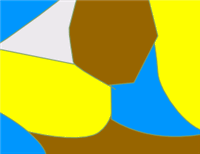# Data Structures- Graph Theory and Coloring

Page 1 of 1

## 0 Replies - 43663 Views - Last Post: 26 May 2011 - 08:26 AMRate Topic://<![CDATA[ rating = new ipb.rating( 'topic_rate_', { url: 'https://www.dreamincode.net/forums/index.php?app=forums&module=ajax&section=topics&do=rateTopic&t=233544&amp;s=4d9b82b7eb6af8a3247c4644999778d9&md5check=' + ipb.vars['secure_hash'], cur_rating: 0, rated: 0, allow_rate: 0, multi_rate: 1, show_rate_text: true } ); //]]>

### #1 macosxnerd101•• Games, Graphs, and Auctions
•Reputation: 12742
• Posts: 45,926
• Joined: 27-December 08

# Data Structures- Graph Theory and Coloring

Posted 26 May 2011 - 08:26 AM

This tutorial will introduce coloring in Graph Theory, including vertex, edge, and face coloring; the Four-Color theorem, and applications of graph coloring.

When dealing with Graph coloring, regardless of the surface (vertex, edge, face), no two colors can be adjacent to each other. While this is fairly simple, graph coloring provides a lot of information that can be very helpful later on.

Vertex Coloring
With vertices, they are considered adjacent if they are connected by the same edge. The goal when coloring a graph's vertices is to find the minimum number of distinct colors required to satisfy the condition that no two adjacent vertices have the same color. This number of colors is called the chromatic number of a graph, and is represented by a chi. Some graphs have very formulaic chromatic numbers, and these are good to know. Complete graphs (Kn) always have a chromatic number Chi(n), since all the vertices are adjacent to one another. All bipartite graphs (even complete bipartite graphs) have a chromatic number Chi(2), since none of the vertices are adjacent to other vertices in the sets they are located in. Cyclic graphs (Cn) have a chromatic number of Chi(2) or Chi(3), depending on whether n is even or odd. If n is even, the chromatic number is Chi(2). If it is odd, the chromatic number is Chi(3). Below is an example of a graph, specifically the Peterson graph, colored on its vertices. Its chromatic number is Chi(3).Edge Coloring
Edge coloring works basically the same as vertex coloring, with the difference being that no two edges incident to the same vertex can have the same color. The chromatic number for edges is called the chromatic index, and is denoted by a chi'(n) (pronounced chi prime). While edge coloring is pretty simple, it has a lot of properties that are important to understand. Vizing's theorem discusses one of these properties in the context of simple graphs. It states that the chromatic index for a simple graph is either the maximum degree or one greater. Because no two edges are connected to the same pair of vertices, there can be no more than the maximum degree of edges attached to any single vertex. Thus, disjoint edges can have the same coloring. Expanding to multigraphs and hypergraphs, edge coloring can be no less than the maximum degree of the graph. In the case of a bipartite graph, the chromatic index is exactly equal to the maximum degree. This is called Konig's bipartite theorem.

Another property based on edge coloring is that the chromatic index of a matching graph, a graph where the maximum degree is one, is also one. Because no two edges are incident to the same vertex, there will be no conflicts with edge coloring.

Applications of Vertex and Edge Coloring
Vertex and edge colorings have numerous applications. One such application is determining the viability of two graphs for possibly being isomorphic. In other words, based on vertex and edge colorings on two graphs, it is possible to determine if they are not isomorphic. This is done first by comparing the chromatic numbers and indices of the two graphs. If they are not equal, the graphs are not isomorphic. If they are equal, then the coloring patterns of the two graphs are examined. While chromatic numbers and indices provide a lot of information, it is possible for two graphs to have the same values for these characteristics, but have different coloring patterns. So based on the coloring pattern, two graphs are considered non-isomoprhic if their coloring patterns are not the same.

Another application of vertex and edge coloring is the ability to partition these elements into sets based on common characteristics. For example, in a zoo, only animals that will not harm each other can be stored in the same habitat. So setting up a graph where each animal is a vertex, and the edges connect them to the other animals with which they cannot share a habitat. Vertices with the same colors represent animals that can be stored together. The more optimal the coloring, the fewer habitats are needed.

Four-Color Theorem
The four-color theorem deals with planar graphs. It states that the faces of any planar graph can be colored with no more than four colors. The plane surrounding the graph is also considered a face. As with edge and vertex coloring, no two adjacent faces can have the same color. Below is a planar graph with its faces colored. One benefit of the four color theorem is that it can be used as one check to see if a graph is planar, which may sometimes be more efficient than checking for instances of K5 or K3,3 within the graph. If the graph requires more than four colors for its faces, it cannot be planar.Is This A Good Question/Topic? 0

Page 1 of 1

 .related ul { list-style-type: circle; font-size: 12px; font-weight: bold; } .related li { margin-bottom: 5px; background-position: left 7px !important; margin-left: -35px; } .related h2 { font-size: 18px; font-weight: bold; } .related a { color: blue; }